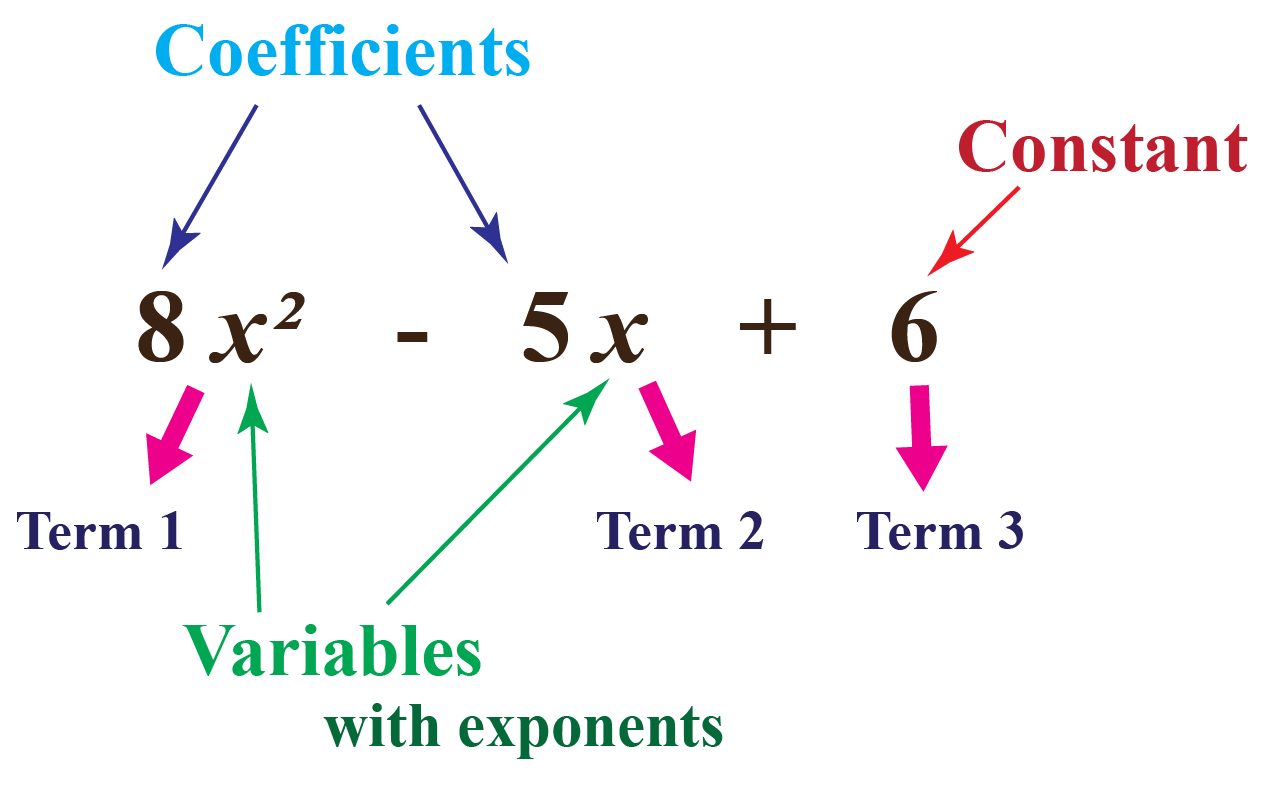# Types of Polynomials

Types of Polynomials
Go back to  'Polynomials'

We have been studying polynomials from a very young age.

When we were first told about variables and expressions, we were simply dealing with polynomials.

For example, if Mathew has $$x$$ pencils and if John has 2 more pencils than Mathew, then John must have $$x + 2$$ pencils.

$$x + 2$$ is a polynomial.But do you know that polynomials can be classified into different types?

For example, Mathew has $$x$$ pencils, which is a monomial and John has $$x+2$$ pencils, which is a binomial.

In this mini-lesson, we will explore the world of polynomials by learning about different types of polynomials through interesting simulations, some solved examples, and a few interactive questions for you to test your understanding.

Let's begin!

## Lesson Plan

 1 What Are the Types of Polynomials Based on Degrees? 2 Tips and Tricks 3 Important Notes on Types of Polynomials 4 Solved Examples on Types of Polynomials 5 Interactive Questions on Types of Polynomials

## What Are Polynomials?

Polynomials are expressions with one or more terms having a non-zero coefficient.

These terms comprise variables, exponents, and constants.

A standard polynomial is one where the highest degree is the first term, and the subsequent terms are arranged in ascending order based on the powers or the exponents.

Polynomials are classified based on the number of terms present in the expression as a monomial, binomial, and trinomial, which we will see in detail under types of polynomials.

Other terms related to polynomials are constant, coefficient, and degree of the polynomial.

The first term of the polynomial is called the leading term.

The number without any variable is called a constant.

The number preceding a variable is called a coefficient.

The power of the leading term or the highest power of the variable is called the degree of the polynomial.## What Are the Types of Polynomials Based on Degrees?

Based on the degree of the polynomial, polynomials can be classified into 4 major types:

• Zero or Constant polynomial
• Linear polynomial
• Cubic polynomial

Look at the table given below to understand the meaning of the types of polynomials with examples.

 Type of polynomial Meaning Examples Zero or constant polynomial Polynomials with 0 degrees are called zero polynomials. $$3\ or\ 3x^0$$ $$56\ or\ 56y^0$$ $$8\ or\ 8z^0$$ Linear polynomial Polynomials with 1 as the degree of the polynomial are called linear polynomials. In linear polynomials, the highest exponent of the variable(s) is 1 $$x+y-4$$ $$5m+7n$$ $$2p$$ Quadratic polynomial Polynomials with 2 as the degree of the polynomial are called quadratic polynomials. $$8x^2+7y-9$$ $$m^2+mn-6$$ $$2p^2-7$$ Cubic polynomial Polynomials with 3 as the degree of the polynomial are called cubic polynomials. $$3x^3$$ $$6m^3-mn+n^2-4$$ $$p^3+pqr-7$$Tips and Tricks
1. Before learning about the types of polynomials, it is important to learn what do we consider as polynomials.
• Polynomials should have a whole number as the degree. Expressions with negative exponents are not polynomials. For example, $$x^{-2}$$ is not a polynomial.
• Polynomials do not have variables in their denominator. For example, $$\dfrac{2}{x+2}$$ is not a polynomial.

## What Are the Types of Polynomials Based on Terms?

There are different types of polynomials. Based on the number of terms, polynomials are classified as:

• Monomials
• Binomials
• Trinomials

A monomial is a polynomial expression that contains only one term.

For example, $$2x + 5x + 10x$$ is a monomial because when we add the like terms it results in $$17x$$.

Furthermore, $$4t$$, $$21x$$, $$2y$$, $$9pq$$ are also monomials.

A binomial is a polynomial with two, unlike terms.

For example, $$3x + 4x^2$$ is a binomial as it contains two unlike terms, that is, $$3x$$ and $$4x^2$$.

Likewise, $$10pq + 13p^2$$ is also a binomial.

A trinomial is a polynomial with three, unlike terms.

For example, $$3x + 5x^2 – 6x^3$$ is a trinomial.

Likewise, $$12pq + 4x^2 – 10$$ is also a trinomial.

We can be classifying polynomials based on the number of terms into 3 types.

But, we can also have more than 3 terms in the polynomials.

Polynomials that have 4 unlike terms are called four-term polynomials.

Similarly, polynomials with 5 terms are called five-term polynomials, and so on.

More Important Topics
Numbers
Algebra
Geometry
Measurement
Money
Data
Trigonometry
Calculus